Courses

# ICAI Notes 2.2 - Theory of Consumer Behaviour CA Foundation Notes | EduRev

## CA Foundation : ICAI Notes 2.2 - Theory of Consumer Behaviour CA Foundation Notes | EduRev

The document ICAI Notes 2.2 - Theory of Consumer Behaviour CA Foundation Notes | EduRev is a part of the CA Foundation Course Economics for CA CPT.
All you need of CA Foundation at this link: CA Foundation

Learning Objectives

• know the meaning of utility
• understand how consumers try to maximize their satisfaction by spending on different goods.

The demand for a commodity depends on the utility of that commodity to a consumer. If a consumer gets more utility from a commodity, he would be willing to pay a higher price and vice-versa.

2.0 WHAT IS UTILITY?

All desires, tastes and motives of human beings are called wants in Economics. Wants may arise due to elementary and psychological causes. Since, the resources are limited; he has to choose between urgent wants and not so urgent wants.

All wants of human beings exhibit some characteristic features.

1. Wants are unlimited

2. Every wants is satiable

3. Wants are competitive

4. Wants are complementary

5. Wants are alternative

6. Wants vary with time, place, and person

7. Some wants recur again

9. Wants become habits and customs In Economics wants are classified into three categories, viz., necessaries, comforts and luxuries.

Necessaries: Necessaries are those which are essential for living. Man cannot do well with barest necessaries of life alone. He requires some more necessaries to keep him fit for taking up productive activities. These are called necessaries of efficiency. There is another type of necessaries are called conventional necessaries. By custom and tradition, people require some wants to be satisfied.

Comforts: Comforts refer to those goods and services which are not essential for living but which are required for a happy living. It lies between the ‘necessaries’ and ‘luxuries’.

Luxuries: Luxuries are those wants which are superfluous and expensive. They are not essential for living, however, they may add efficiency to the consumer. Utility is the want satisfying power of a commodity. It is a subjective entity and varies from person to person. It should be noted that utility is not the same thing as usefulness. Even  harmful things like liquor, may be said to have utility from the economic stand point because people want them. Thus in Economics, the concept of utility is ethically neutral.

Utility: Utility is an anticipated satisfaction by the consumer, and the satisfaction is the actual satisfaction derived. Utility hypothesis forms the basis for the theory of consumer’s behaviour. From time to time different theories have been advanced to explain consumer’s behaviour and thus to explain his demand for the product. Two important theories are (i) Marginal Utility Analysis propounded by Marshall, and (ii) Indifference Curve Analysis propounded by Hicks and Allen.

2.1 MARGINAL UTILITY ANALYSIS

This theory which is formulated by Alfred Marshall, a British economist, seeks to explain how a consumer spends his income on different goods and services so as to attain maximum satisfaction. This theory is based on certain assumptions. But before stating the assumptions, let us understand the meaning of total utility and marginal utility. Total Utility is otherwise known as “Full Satiety”. Marginal Utility is also known as marginal satiety.

Total utility : It is the sum of the utility derived from an different units of a commodity consumed by a consumer.

Marginal utility : It is the additional utility derived from additional unit of a commodity.

2.1.0 Assumptions of Marginal Utility Analysis

(1) The Cardinal Measurability of Utility : According to this theory, utility is a cardinal concept i.e., utility is a measurable and quantifiable entity. Thus a person can say that he derives utility equal to 10 units from the consumption of 1 unit of commodity A and 5 from the consumption of 1 unit of commodity B. Since, he can express his satisfaction quantitatively, he can easily compare different commodities and express which commodity gives better utility or satisfaction and by how much. According to this theory, money is the measuring rod of utility. The amount of money which a person is prepared to pay for a unit of a good rather than go without it is a measure of the utility which he derives from the good.

(2) Constancy of the Marginal Utility of Money : The marginal utility of money remains constant throughout when the individual is spending money on a good. This assumption although not realistic, has been made in order to facilitate the measurement of utility of commodities in terms of money.

(3) The Hypothesis of Independent Utility : The total utility which a person gets from the whole collection of goods purchased by him is simply the sum total of the separate utilities of the goods. The theory ignores complementarity between goods.

2.1.1 The Law of Diminishing Marginal Utility

One of the important laws under Marginal Utility analysis is the Law of Diminishing Marginal Utility. The law of diminishing marginal utility is based on an important fact that while total wants of a person are virtually unlimited, each single want is satiable i.e., each want is capable of being satisfied.

Since each want is satiable, as a consumer consumes more and more units of a good, the intensity of his want for the good goes on decreasing and a point is reached where the consumer no longer wants it.

Marshall who was the exponent of the marginal utility analysis stated the law as follows :

“The additional benefit which a person derives from a given increase in stock of a thing diminishes with every increase in the stock that he already has.”

This law describes a very fundamental tendency of human nature. In simple words it says that as a consumer takes more units of a good, the extra satisfaction that he derives from an extra unit of a good goes on falling. It is to be noted that it is the marginal utility and not the total utility which declines with the increase in the consumption of a good.

Table 5 : Total and marginal utility schedules

 Quantity of tea consumed  (cups per day) Total utility Marginal utility 1 30 30 2 50 20 3 65 15 4 75 10 5 83 8 6 89 6 7 93 4 8 96 3 9 98 2 10 99 0 11 95 -4

Let us illustrate the law with the help of an example. Consider Table 5, in which we have presented the total utility and marginal utility derived by a person from cups of tea consumed per day. When one cup of tea is taken per day, the total utility derived by the person is 30 utils (unit of utility) and marginal utility derived is also 30 utils with the consumption of 2nd cup per day the total utility rises to 50 but marginal utility falls to 20. We see as the consumption of tea increases to 10 cups per day, marginal utility from the additional cups goes on diminishing (i.e., the total utility goes on increasing at a diminishing rate). However, when the cups of tea consumed per day increases to 11, then instead of giving positive marginal utility, the eleventh cup gives negative marginal utility because it may cause him sickness.

We have graphically represented the data of the above table in Figure 13. Graphically we can represent the relationship between the total utility and marginal utility.

From the above diagram we can conclude the three important relationships between total utility and marginal utility
1. When the total utility rises the marginal utility diminishes.
2. When the total utility is maximum then the marginal utility is zero.
3. When the total utility is diminishing then the marginal utility is negative.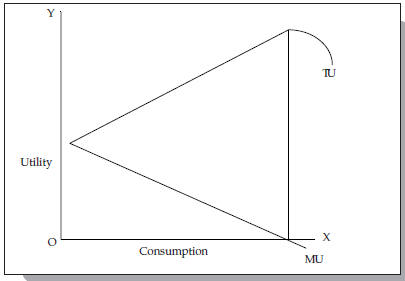Fig. 12 : Total Utility and Marginal Utility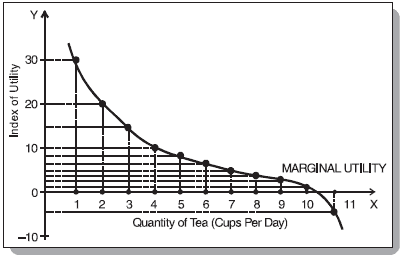Fig. 13 : Marginal utility of tea consumed

As will be seen from the figure, the marginal utility curve goes on declining throughout. The diminishing marginal utility curve applies almost to all commodities. A few exceptions however, have been pointed out by some economists. According to them, this law does not apply to money, music and hobbies. While this may be true in initial stages, beyond a certain limit these will also be subjected to diminishing utility.

Limitations of the Law

The law of diminishing marginal utility is applicable only under certain assumptions.

(i) The different units consumed should be identical in all respects. The habit, taste, treatment and income of the consumer also remain unchanged.

(ii) The different units consumed should consist of standard units. If a thirsty man is given water by successive spoonfuls, the utility of second spoonful may conceivably be greater than the utility of the first.

(iii) There should be no time gap or interval between the consumption of one unit and another unit i.e. there should be continuous consumption.

(iv) The law may not apply to articles like gold, cash where a greater quantity may increase the lust for it.

(v) The shape of the utility curve may be affected by the presence or absence of articles which are substitutes or complements. The utility obtained from tea may be seriously affected if no sugar is available.

2.1.2 Consumer’s Surplus : The concept of consumer’s surplus was evolved by Alfred Marshall. This concept occupies an important place not only in economic theory but also in economic policies of government and decision-making of monopolists.

It has been seen that consumers generally are ready to pay more for the goods than they actually pay for them. This extra satisfaction which consumers get from their purchase of goods is called by Marshall as consumer’s surplus.

Marshall defined the concept of consumer’s surplus as “excess of the price which a consumer would be willing to pay rather than go without a thing over that which he actually does pay, is the economic measure of this surplus satisfaction........it may be called consumer’s surplus”.

Thus consumer’s surplus = What a consumer is ready to pay - What he actually pays.

The concept of consumer’s surplus is derived from the law of diminishing marginal utility. As we know from the law of diminishing marginal utility, the more of a thing we have, the lesser marginal utility it has. In other words, as we purchase more of a good, its marginal utility goes on diminishing. The consumer is in equilibrium when marginal utility is equal to given price i.e., he purchases that many number of units of a good at which marginal utility is equal to price (It is assumed that perfect competition prevails in the market). Since the price is fixed for all the units of the good he purchases except for the one at margin, he gets extra utility; this extra utility or extra surplus for the consumer is called consumer’s surplus.

Prof. Hicks has redefined the concepts as the money income gained by a man arising from a fall in price of goods he purchases.

It is often argued that this concept is a theoretical toy. The surplus satisfaction cannot be measured precisely. In case of very essential goods of life; utility is very high but prices paid of them are low giving rise to infinite surplus satisfaction. Further, it is difficult to measure the marginal utilities of different units of a commodity consumed by person.

Consider Table 6 in which we have illustrated the measurement of consumer’s surplus in case of commodity X. The price of X is assumed to be Rs. 20.

Table 6 : Measurement of Consumer’s Surplus

 No. of units Marginal Utility Price (Rs.) Consumer’s Surplus 1 30 20 10 2 28 20 8 3 26 20 6 4 24 20 4 5 22 20 2 6 20 20 0 7 18 20 -

We see from the above table that when consumer’s consumption increases from 1 to 2 units, his marginal utility falls from Rs. 30 to Rs. 28. His marginal utility goes on diminishing as he increases his consumption of good X. Since marginal utility for a unit of good indicates the price the consumer is willing to pay for that unit, and since price is assumed to be fixed at Rs. 20, the consumer enjoys a surplus at every unit of purchase above 6 units. Thus when the consumer is purchasing 1 unit of X, the marginal utility is worth Rs. 30 and price fixed is Rs. 20, thus he is deriving a surplus of Rs. 10. Similarly when he purchases 2 units of X, he enjoys a surplus of Rs. 8 [Rs. 28 – Rs. 20]. This continues and he enjoys consumer’s surplus equal to Rs. 6, 4, 2 respectively from 3rd, 4th and 5th unit. When he buys 6 units, he is in equilibrium because here his marginal utility is equal to the market price or he is willing to pay a sum equal to the actual market price.

Here he enjoys no surplus. Thus, given the price of Rs. 20 per unit, the total surplus which the consumer will get, is Rs. 10 + 8 + 6 + 4 + 2 + 0 = 30. The concept of consumer’s surplus can also be illustrated graphically. Consider figure 10. On the Xaxis is measured the amount of the commodity and on the Y-axis the marginal utility and the price of the commodity. MU is the marginal utility curve which slopes downwards, indicating that as the consumer buys more units of the commodity, its marginal utility falls. Marginal utility shows the price which a person is willing to pay for the different units rather than go without them.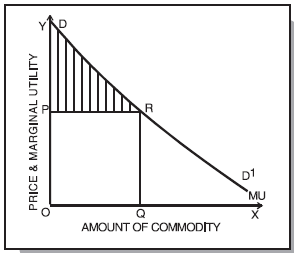Fig. 14 : Marshall’s Measure of Consumer’s Surplus

without them. If OP is the price that prevails in the market, then consumer will be in equilibrium when he buys OQ units of the commodity, since at OQ units, marginal utility is equal to the given price OP. The last unit, i.e., Qth unit does not yield any consumer’s surplus because here price paid is equal to the marginal utility of the Qth unit. But for units before Qth unit, marginal utility is greater than the price and thus these units fetch consumer’s surplus to the consumer.

In Figure 14, the total utility is equal to the area under the marginal utility curve up to point Q i.e. ODRQ. But given the price equal to OP, the consumer actually pays OPRQ. The consumer derives extra utility equal to DPR which is nothing but consumer’s surplus.

Limitations :

(1) Consumer’s surplus cannot be measured precisely - because it is difficult to measure the marginal utilities of different units of a commodity consumed by a person.

(2) In the case of necessaries, the marginal utilities of the earlier units are infinitely large. In such case the consumer’s surplus is always infinite.

(3) The consumer’s surplus derived from a commodity is affected by the availability of substitutes.

(4) There is no simple rule for deriving the utility scale of articles which are used for their prestige value (e.g., diamonds).

(5) Consumer’s surplus cannot be measured in terms of money because the marginal utility of money changes as purchases are made and the consumer’s stock of money diminishes. (Marshall assumed that the marginal utility of money remains constant. But this assumption is unrealistic).

(6) The concept can be accepted only if it is assumed that utility can be measured in terms of money or otherwise. Many modern economists believe that this cannot be done.

2.2 INDIFFERENCE CURVE ANALYSIS

In the last section we discussed marginal utility analysis of demand. A very popular alternative and more realistic method of explaining consumer’s demand is the Indifference Curve Analysis. This approach to consumer behaviour is based on consumer preferences. It believes that human satisfaction being a psychological phenomenon cannot be measured quantitatively in monetary terms as was attempted in Marshall’s utility analysis. In this approach it is felt that it is much easier and scientifically more sound to order preferences than to measure them in terms of money. The consumer preference approach, is, therefore an ordinal concept based on ordering of preferences compared with Marshall’s approach of cardinality.

2.2.0 Assumptions Underlying Indifference Curve Approach

(i) The consumer is rational and possesses full information about all the relevant aspects of economic environment in which he lives.

(ii) The consumer is capable of ranking all conceivable combinations of goods according to the satisfaction they yield. Thus if he is given various combinations say A, B, C, D, E he can rank them as first preference, second preference and so on. If a consumer happens to prefer A to B, he can not tell quantitatively how much he prefers A to B.

(iii) If the consumer prefers combination A to B, and B to C, then he must prefer combination A to C. In other words, he has a consistent consumption pattern behaviour. (iv) If combination A has more commodities than combination B, then A must be preferred to B.

2.2.1 What are Indifference Curves?

Ordinal analysis of demand (here we will discuss the one given by Hicks and Allen) is based on indifference curves. An indifference curve is a curve which represents all those combinations of goods which give same satisfaction to the consumer. Since all the combinations on an indifference curve give equal satisfaction to the consumer, the consumer is indifferent among them. In other words, since all the combinations provide same level of satisfaction the consumer prefers them equally and does not mind which combination he gets.

To understand indifference curves let us consider the example of a consumer who has one unit of food and 12 units of clothing. Now we ask the consumer how many units of clothing he is prepared to give up to get an additional unit of food, so that his level of satisfaction does not change. Suppose the consumer says that he is ready to give up 6 units of clothing to get an additional unit of food. We will have then two combinations of food and clothing giving equal satisfaction to consumer : Combination A has 1 unit of food and 12 units of clothing, combination B has 2 units of food and 6 units of clothing. Similarly, by asking the consumer further how much of clothing he will be prepared to forgo for successive increments in his stock of food so that his level of satisfaction remains unaltered, we get various combinations as given below :

Table 7 : Indifference Schedule

 Combination Food Clothing MRS A 1 12 B 2 6 6 C 3 4 2 D 4 3 1

Now if we draw the above schedule we will get the following figure.

In Figure 15, an indifference curve IC is drawn by plotting the various combinations of the indifference schedule. The quantity of food is measured on the X axis and the quantity of clothing on the Y axis. As in indifference schedule, combinations lying on an indifference curve will give the consumer same level of satisfaction.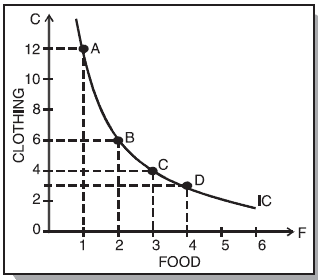Fig. 15 : A Consumer’s Indifference Curve

2.2.2 Indifference Map :

A set of indifference curves is called indifference map. An indifference map depicts complete picture of consumer’s tastes and preferences. In Figure 16, an indifference map of a consumer is shown which consists of three indifference curves. We have taken good X on X-axis and good Y on Y-axis. It should be noted that while the consumer is indifferent among the combinations lying on the same indifference curve, he certainly prefers the combinations on the higher indifference curve to the combinations lying on a lower indifference curve because a higher indifference curve signifies a higher level of satisfaction. Thus while all combinations of IC1 give same satisfaction, all combinations lying on IC2 give greater satisfaction than those lying on IC1.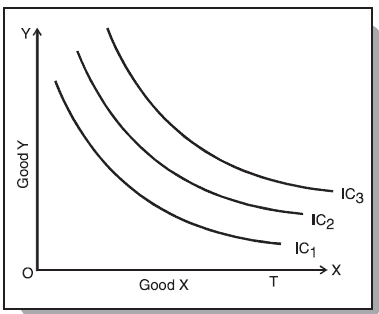Fig. 16 : Indifference Map

2.2.3. Marginal Rate of Substitution : Marginal Rate of Substitution (MRS) is the rate at which the consumer is prepared to exchange goods X and Y. Consider Table-7. In the beginning the consumer is consuming 1 unit of food and 12 units of clothing. Subsequently, he gives up 6 units of clothing to get an extra unit of food, his level of satisfaction remaining the same. The MRS here is 6. Like wise which he moves from B to C and from C to D in his indifference schedule, the MRS are 2 and 1 respectively. Thus, we can define MRS of X for Y as the amount of Y whose loss can just be compensated by a unit gain of X in such a manner that the level of satisfaction remains the same. We notice that MRS is falling i.e., as the consumer has more and more units of food, he is prepared to give up less and less units of cloths. There are two reasons for this.

1. The want for a particular good is satiable so that when a consumer has its more quantity, his intensity of want for it decreases. Thus, when consumer in our example, has more units of food, his intensity of desire for additional units of food decreases.

2. Most of the goods are imperfect substitutes of one another. If they could substitute one another perfectly. MRS would remain constant.

2.2.4 Properties of Indifference Curves : The following are the main characteristics or properties of indifference curves :

(i) Indifference curves slope downward to the right : This property implies that when the amount of one good in combination is increased, the amount of the other good is reduced. This is essential if the level of satisfaction is to remain the same on an indifference curve.

(ii) Indifference curves are always convex to the origin : It has been observed that as more and more of one commodity (X) is substituted for another (Y), the consumer is willing to part with less and less of the commodity being substituted (i.e. Y). This is called diminishing marginal rate of substitution. Thus in our example of food and clothing, as a consumer has more and more units of food, he is prepared to forego less and less units of clothing. This happens mainly because want for a particular good is satiable and as a person has more and more of a good, his intensity of want for that good goes on diminishing. This diminishing marginal rate of substitution gives convex shape to the indifference curves. However, there are two extreme situations. When two goods are perfect substitutes of each other, the indifference curve is a straight line on which MRS is constant. And when two goods are perfect complementary goods (e.g. gasoline and water in a car), the indifference curve will consist of two straight line with a right angle bent which is convex to the origin or in other words, it will be L shaped.

(iii) Indifference curves can never intersect each other : No two indifference curves will intersect each other although it is not necessary that they are parallel to each other. In case of intersection the relationship becomes logically absurd because it would show that higher and lower levels are equal which is not possible. This property will be clear from the following Figure 17.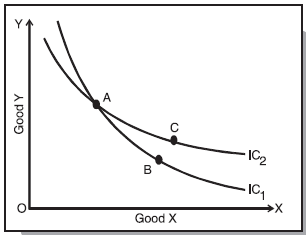Fig. 17 : Intersecting Indifference Curves

In figure 17, IC1 and IC2 intersect at A. Since A and B lie on IC1, they give same satisfaction to the consumer. Similarly since A and C lie on IC2, they give same satisfaction to the consumer. This implies that combination B and C are equal in terms of satisfaction. But a glance will show that this is an absurd conclusion because certainly combination C is better than combination B because it contains more units of commodities X and Y. Thus we see that no two indifference curves can touch or cut each other.

(iv) A higher indifference curve represents a higher level of satisfaction than the lower indifference curve : This is because combinations lying on a higher indifference curve contain more of either one or both goods and more goods are preferred to less of them.

(v) Indifference curve will not touch the axis : Another characteristic feature of indifference curve is that it will not touch the X axis or Y axis. This is born out of our assumption that the consumer is considering different combination of two commodities. If an indifference curve touches the Y axis at a point P as shown in the figure 18 it means that the consumer is satisfied with OP units of y commodity and zero units of x commodity. This is contrary to our assumption that the consumer wants both commodities although in a smaller or larger quantities. Therefore the indifference curve will not touch either the X axis or Y axis.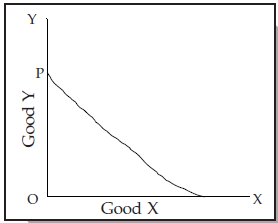Fig. 18 : Indifference Curve

2.2.5 Budget Line : A higher indifference curve shows a higher level of satisfaction than a lower one. Therefore, a consumer in his attempt to maximise satisfaction will try to reach the highest possible indifference curve. But in his pursuit of buying more and more goods and thus obtaining more and more satisfaction he has to work under two constraints : firstly, he has to pay the prices for the goods and, secondly, he has a limited money income with which to purchase the goods.

These constraints are explained by budget line or price line. In simple words a budget line shows all those combinations of two goods which the consumer can buy spending his given money income on the two goods at their given prices. All those combinations which are within the reach of the consumer (assuming that he spends all his money income) will lie on the budget line.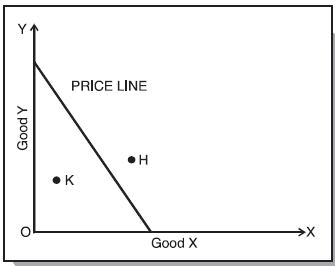Fig. 19 : Price Line

It should be noted that any point outside the given price line, like H, will be beyond the reach of the consumer and any combination lying within the line, like K, shows under spending by the consumer.

2.2.6 Consumer’s Equilibrium : Having explained indifference curves and budget line, we are in a position to explain how a consumer reaches equilibrium position. A consumer is in equilibrium when he is deriving maximum possible satisfaction from the goods and is in no position to rearrange his purchases of goods. We assume that : (i) the consumer has a given indifference map which shows his scale of preferences for various combinations of two goods X and Y. (ii) he has a fixed money income which he has to spend wholly on goods X and Y. (iii) prices of goods X and Y are given and are fixed for him. (iv) All goods are homogeneous and divisible. (v) The consumers acts ‘rationally’ and maximizes his satisfaction.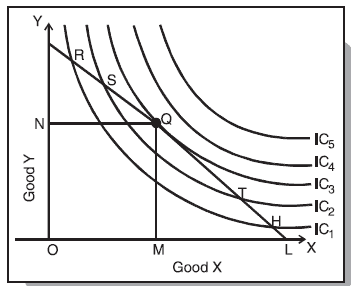Fig. 20 : Consumer’s Equilibrium

To show which combination of two goods X and Y the consumer will buy to be in equilibrium we bring his indifference map and budget line together.

We know by now, that the indifference map depicts the consumer’s preference scale between various combinations of two goods and the budget line shows various combinations which he can afford to buy with his given money income and prices of the two goods. Consider Figure 20, in which IC1, IC2, IC3, IC4 and IC5 are shown together with budget line PL for good X and good Y. Every combination on budget line PL costs the same. Thus combinations R, S, Q, T and H cost the same to the consumer. The consumer’s aim is to maximise his satisfaction and for this he will try to reach highest indifference curve.

But since there is a budget constraint he will be forced to remain on the given budget line, that is he will have to choose any combinations from among only those which lie on the given price line.

Which combination will he choose? Suppose he chooses R, but we see that R lies on a lower indifference curve IC1, when he can very well afford S, Q or T lying on higher indifference curve. Similar is the case for other combinations on IC1, like H. Again, suppose he chooses combination S (or T) lying on IC2. But here again we see that the consumer can still reach a higher level of satisfaction remaining within his budget constraints i.e., he can afford to have combination Q lying on IC3 because it lies on his budget line. Now what if he chooses combination Q? We find that this is the best choice because this combination lies not only on his budget line but also puts him on highest possible indifference curve i.e., IC3. The consumer can very well wish to reach IC4 or IC5, but these indifference curves are beyond his reach given his money income. Thus the consumer will be at equilibrium at point Q on IC3. What do we notice at point Q? We notice that at this point, his budget line PL is tangent to the indifference curve IC3. In this equilibrium position (at Q), the consumer will buy OM of X and ON of Y.

At the tangency point Q, the slopes of the price line PL and indifference curve IC3 are equal. The slope of the indifference curve shows the marginal rate of substitution of X for Y (MRSxy) which is equal to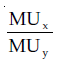while the slope of the price line indicates the ratio between the prices of two goods i.e.,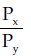At equilibrium point Q,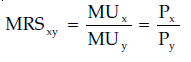ratio between the prices of the two goods.

Thus, we can say that the consumer is in equilibrium position when price line is tangent to the indifference curve or when the marginal rate of substitution of goods X and Y is equal to the ratio between the prices of the two goods.

SUMMARY

The theory of consumer’s behaviour seeks to explain the determination of consumer’s equilibrium. Two famous approaches to consumer’s equilibrium are (i) Marginal Utility Analysis (ii) Indifference Curve Analysis.

Marginal utilility analysis is framed within the parameters of two laws : Law of diminishing marginal utility and the law of equi-marginal utility. The law of diminishing marginal utility states that as a consumer increases the consumption of a commodity, every successive unit of the commodity gives lesser and lesser satisfaction to the consumer i.e., marginal utility of the commodity falls.

The indifference curve theory which is an ordinal theory shows the household’s preference between alternative bundles of goods by means of indifference curves. A single curve joins all those combinations of goods which give the household equal satisfaction or utility and between which the household is thus indifferent. The household reaches equilibrium when for a given money income and given market price, it has reached the highest attainable level of satisfaction. At such a point, the budget line is tangent to the indifference curve. At the tangency point, the following condition is satisfied :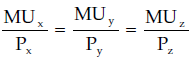The indifference curve analysis is superior to utility analysis : (i) it dispenses with the assumption of measurability of utility (ii) it studies more than one commodity at a time (iii) it does not assume constancy of money (iv) it segregates income effect from substitution effect.

,

,

,

,

,

,

,

,

,

,

,

,

,

,

,

,

,

,

,

,

,

;